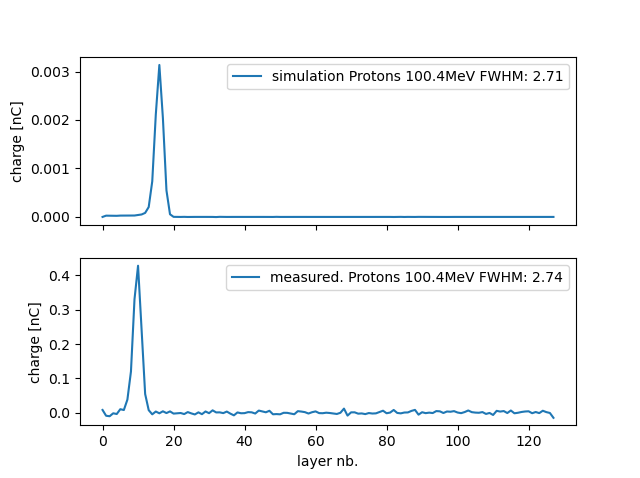# Simulation of a multilayer Faraday Cup

Dear FLUKA experts,

I want to simulate a MLFC. The goal is to predict the charges in the corresponding layers. Therefor I scored ALL-POSItive charges and derived the result. If you compare the simulation with actual measured values, on the one hand you see a shift in the range, which may be due to the imprecise definition of the insolator materials, on the other hand the number of charges in the simulation is far too low.To normalize the fluka-results I multiplied by elementary Charge, 1,4E+10 protons per spill and a factor 5 (there were five measurements during one spill which were added up).Protons_100_4MeV.inp (75.6 KB) .

I would appreciate it if you could help me.
Michael

Dear Michael,

I scored ALL-POSItive charges

Requesting ALL-POSI in your USRBIN implies scoring the fluence of all positively charged particles, not the charge itself. I take that you have numerically differentiated it along z (I did not complete the derivation, but it indeed looks like the gradient of the charged particle fluence is related to the charge density variation, with a minus sign).

You probably started from a 1D Projection in Flair. Note that this returns the average value (not the integral over the transverse plane) of the fluence at a given Z, the average being performed over the surface of the scoring transverse XY plane. Thus, if you double the size of the USRBIN along X and Y, the “1D Projection” value will drop by a factor 4 (!). Most of your normalization issues likely come from forgetting to multiply by the 225 cm^2 of your transverse USRBIN plane. I.e., the plotted quantity in your “1D Projection” still has units of fluence (1/cm^2).

Combining the above items, once you have a numerical estimate of the derivative of the fluence along z (in resulting units of 1/cm^3), you

• flip the sign,
• multiply by 225 cm^2 (you get something in 1/cm),
• multiply by the bin width along Z (you get a dimensionless number, i.e. charge in elementary charge units).
• multiply by the elementary charge (1.602D-19 C),
• convert to nC (multiply by 1.0D9),
• multiply by your number of protons per spill (1.4D10).

I am not convinced you need the factor 5: a MC simulation gives you results per primary incident particle, so once you have scaled with the number of incoming protons, that’s it.

Finally, note that you can score the charge going into each USRBIN bin minus charge going out of each bin using the generalized particle NET-CHRG in the USRBIN card. For this, though, you should use an “X-Y-Z Point” USRBIN type: instead, the usual X-Y-Z type (without “Point”) apportions quantities along bins crossed by the particle step, which is not meaningful for net charge estimates (it’s a locally deposited quantity). Also in this situation the above comment on the “1D Projection” (say Z) average applies: you will get a quantity in 1/cm^3 giving the charge density averaged over XY. To obtain the charge, you have to multiply by the XY surface and then by the Z bin width (as indicated above).

Cesc

Dear Cesc,
thanks a lot for your help, and sorry for the late anwer.
Your advice really helped a lot!Best regards,
Michael